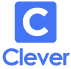https://sbt-test.azurewebsites.net/lesson-plans/motion

# Motion

### Lesson Plans by Oliver Smith

Find this lesson plan and more like it in our Science Category.Motion has been studied by scientists for millennia. Mathematics is often used as the language to describe many things in science, and this is especially true in kinematics, or the study of the motion of objects. Using visual aids to introduce these concepts makes it easier for students to gain a solid understanding and foundation in kinematics that can help them in a range of other activities and industries! The following activities aim to introduce students to graphs and diagrams in a fun and engaging way.

## Student Activities for Motion Include:

(This will start a 2-Week Free Trial - No Credit Card Needed)

(This will start a 2-Week Free Trial - No Credit Card Needed)

## Kinematics / Motion Background Information

Kinematics is an area of study in classical physics that deals with motion. Some people could even argue it is actually an area of Mathematics. We can describe the motion of objects by looking at the different measurable quantities such as displacement, velocity, and acceleration. Displacement is distance with a direction. Velocity, or speed, is how fast something is moving. In order to calculate the average speed you need to know two things: the distance the object has traveled and the time it has taken the object to cover that distance. Science uses the S.I. units for speed, m/s (meters per second). In everyday language, we can also describe speed in the units of mph (miles per hour) or km/h (kilometers per hour). The equation for speed is distance divided by time taken (speed = d ÷ t). Instantaneous speed is the speed at a particular moment, whereas average speed is the mean speed across a large distance. Acceleration is a measure of the rate of change of speed. Acceleration can be positive, meaning velocity is increasing, or negative, meaning velocity is decreasing.

The motion of the object can be described using charts. It is important that students are able to interpret velocity-time graphs and displacement-time graphs. In both of these graphs, time runs along the x-axis with velocity or displacement on the y-axis. For a displacement-time graph, the gradient or slope of the line indicates the direction and the speed an object is traveling. A line with zero gradient (a horizontal line) means the object is not moving. If the line curves, this indicates the object is accelerating, either negatively or positively.

There are two types of quantities in Science: vector quantities and scalar quantities. A vector quantity is a quantity that has both size and direction. Velocity is one example of a vector, where both the magnitude and the direction of the quantity are needed to calculate. Scalar quantities are only measured by magnitude. An example of a scalar quantity is time. Time has no direction, but does have magnitude.

Velocity and acceleration are both vector quantities and can be represented by an arrow. When the acceleration vector is in the same direction as the velocity vector, the object will increase in velocity in that direction. When the acceleration arrow is in the opposite direction to the velocity vector, the object’s velocity will decrease. If there is no acceleration, then the object will travel at a constant velocity; it will not increase or decrease.

(This will start a 2-Week Free Trial - No Credit Card Needed)

Velocity and Acceleration

(This will start a 2-Week Free Trial - No Credit Card Needed)

Be sure to check out the lesson plans for introductory forces too!

## Essential Questions for Motion

1. What is a scalar/vector quantity?
2. How can we describe motion?
3. How can we calculate speed?

### Other Motion Activity Ideas

1. Create a narrative storyboard showing one's motion throughout the day. Make a displacement-time graph to accompany it.
2. Plan an investigation into velocity or displacement using Storyboard That investigation planning resources.
3. Compare different scalar and vector pairs, like velocity and speed, in a T-Chart.

(This will start a 2-Week Free Trial - No Credit Card Needed)

### Pricing

(This will start a 2-Week Free Trial - No Credit Card Needed)

## Looking for More?

Check out the rest of our Lesson Plans!

Our Posters on ZazzleOur Lessons on Teachers Pay Teachershttps://sbt-test.azurewebsites.net/lesson-plans/motion
Explore Our Articles and Examples

### Teacher Resources

Lesson Plans Worksheet TemplatesPoster TemplatesStoryboard Templates